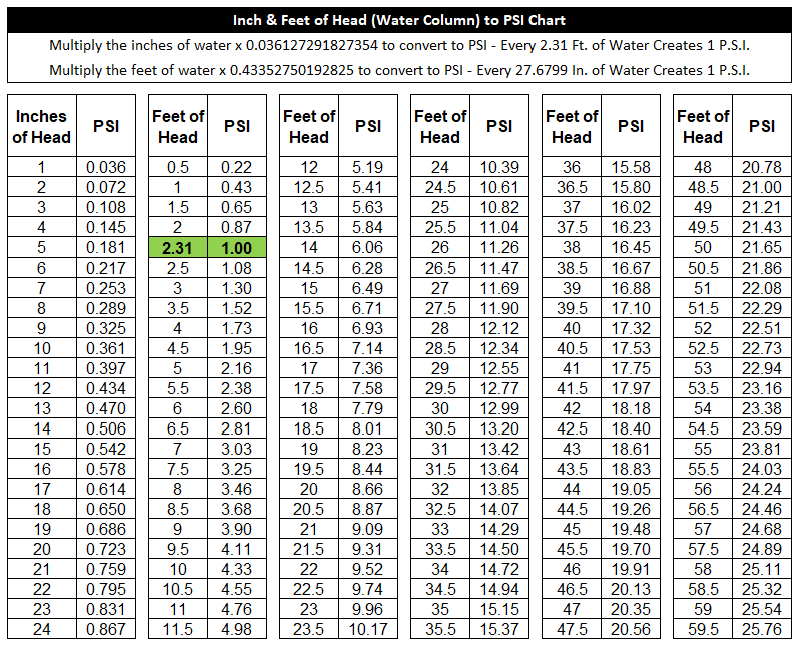Boshart Knowledge Base

# How do I convert Inches or Feet of water to PSI?

To convert Inches & Feet of Head (Water Column) to PSI  use the multipliers below.

Inches of Water to PSI -Multiply the inches of water x 0.036127291827354 to convert to PSI - NOTE: Every 2.3066587368787 Ft. of Water Creates 1 P.S.I.

PSI to Inches of Water - Multiply the PSI x 27.679904842545 to convert to Inches of Water

Feet of Water to PSI - Multiply the feet of water x 0.43352750192825 to convert to PSI - NOTE: Every 27.6799 In. of Water Creates 1 P.S.I.

PSI to Feet of Water - Multiply the PSI x 2.3066587368787 to convert to Feet of Water